# Supervised and Unsupervised Learning in R Programming

• Last Updated : 29 Nov, 2021

Arthur Samuel, a pioneer in the field of artificial intelligence and computer gaming, coined the term “Machine Learning”. He defined machine learning as – “Field of study that gives computers the capability to learn without being explicitly programmed”. In a very layman manner, Machine Learning(ML) can be explained as automating and improving the learning process of computers based on their experiences without being actually programmed i.e. without any human assistance. The process starts with feeding good quality data and then training our machines(computers) by building machine learning models using the data and different algorithms. The choice of algorithms depends on what type of data do we have and what kind of task we are trying to automate. There are mainly four types of learning.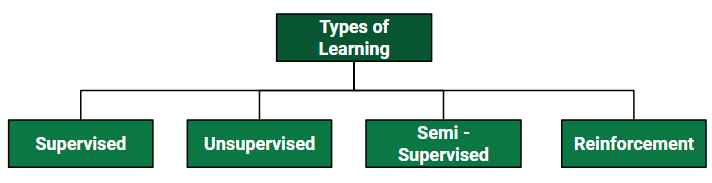In this article let’s discuss the two most important learning e.g Supervised and Unsupervised Learning in R programming

R language is basically developed by statisticians to help other statisticians and developers faster and efficiently with the data. As of now, we know that machine learning is basically working with a large amount of data and statistics as a part of data science the use of R language is always recommended. Therefore, the R language is mostly becoming handy for those working with machine learning making tasks easier, faster, and innovative. Here are some top advantages of the R language to implement a machine learning algorithm in R programming.

### Advantages to Implement Machine Learning Using R Language

• It provides good explanatory code. For example, if you are at the early stage of working with a machine learning project and you need to explain the work you do, it becomes easy to work with R language comparison to python language as it provides the proper statistical method to work with data with fewer lines of code.
• R language is perfect for data visualization. R language provides the best prototype to work with machine learning models.
• R language has the best tools and library packages to work with machine learning projects. Developers can use these packages to create the best pre-model, model, and post-model of the machine learning projects. Also, the packages for R are more advanced and extensive than python language which makes it the first choice to work with machine learning projects.

## Supervised Learning

Supervised learning as the name indicates the presence of a supervisor as a teacher. Basically supervised learning is learning in which we teach or train the machine using data that is well labeled which means some data is already tagged with the correct answer. After that, the machine is provided with a new set of examples(data) so that the supervised learning algorithm analyses the training data(set of training examples) and produces a correct outcome from labeled data. Supervised learning is classified into two categories of algorithms:

• Classification: A classification problem is when the output variable is a category, such as “Red” or “blue” or “disease” and “no disease”.
• Regression: A regression problem is when the output variable is a real value, such as “dollars” or “weight”.

Supervised learning deals with or learns with “labeled” data. This implies that some data is already tagged with the correct answer.

### Implementation in R

Let’s implement one of the very popular Supervised Learning i.e Simple Linear Regression in R programming. Simple Linear Regression is a statistical method that allows us to summarize and study relationships between two continuous (quantitative) variables. One variable denoted x is regarded as an independent variable and the other one denoted y is regarded as a dependent variable. It is assumed that the two variables are linearly related. Hence, we try to find a linear function that predicts the response value(y) as accurately as possible as a function of the feature or independent variable(x). The basic syntax for regression analysis in R is:

Syntax:

lm(Y ~ model)

where,

Y is the object containing the dependent variable to be predicted and model is the formula for the chosen mathematical model.

The command lm() provides the model’s coefficients but no further statistical information. The following R code is used to implement simple linear regression.

Example:

## R

 `# Simple Linear Regression``# Importing the dataset``dataset = ``read.csv``(``'salary.csv'``)` `# Splitting the dataset into the``# Training set and Test set``install.packages``(``'caTools'``)``library``(caTools)``split = ``sample.split``(dataset\$Salary,``                     ``SplitRatio = 0.7)``trainingset = ``subset``(dataset, split == ``TRUE``)``testset = ``subset``(dataset, split == ``FALSE``)` `# Fitting Simple Linear Regression``# to the Training set``lm.r = ``lm``(formula = Salary ~ YearsExperience,``                          ``data = trainingset)``coef``(lm.r)` `# Predicting the Test set results``ypred = ``predict``(lm.r, newdata = testset)` `install.packages``(``"ggplot2"``)``library``(ggplot2)` `# Visualising the Training set results``ggplot``() + ``geom_point``(``aes``(x = trainingset\$YearsExperience,``                          ``y = trainingset\$Salary),``                          ``colour = ``'red'``) +``           ``geom_line``(``aes``(x = trainingset\$YearsExperience,``                         ``y = ``predict``(lm.r, newdata = trainingset)),``                         ``colour = ``'blue'``) +``        ` `ggtitle``(``'Salary vs Experience (Training set)'``) +``xlab``(``'Years of experience'``) +``ylab``(``'Salary'``)` `# Visualising the Test set results``ggplot``() + ``geom_point``(``aes``(x = testset\$YearsExperience,``                          ``y = testset\$Salary),``                          ``colour = ``'red'``) +``           ``geom_line``(``aes``(x = trainingset\$YearsExperience,``                         ``y = ``predict``(lm.r, newdata = trainingset)),``                         ``colour = ``'blue'``) +` `ggtitle``(``'Salary vs Experience (Test set)'``) +``xlab``(``'Years of experience'``) +``ylab``(``'Salary'``)`

Output:

```Intercept YearsExperience
24558.39 10639.23```

Visualizing the Training set results: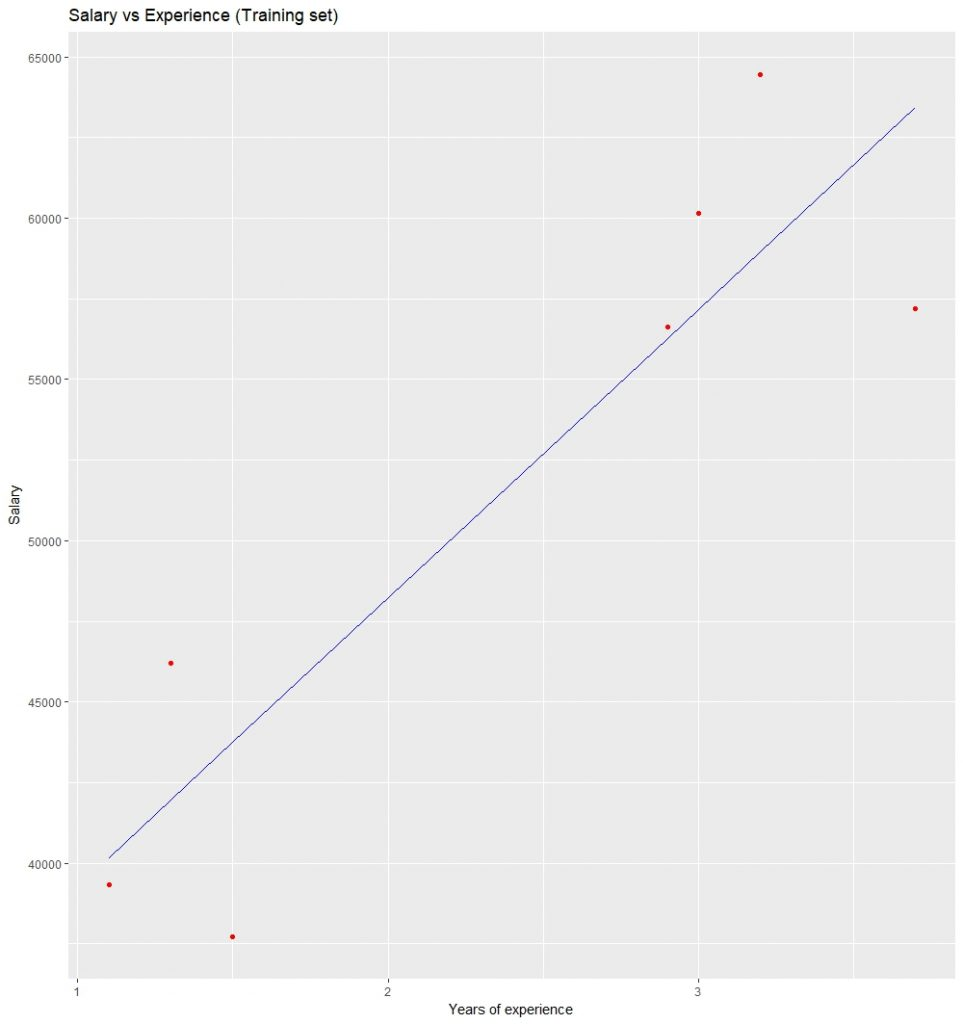Visualizing the Testing set results: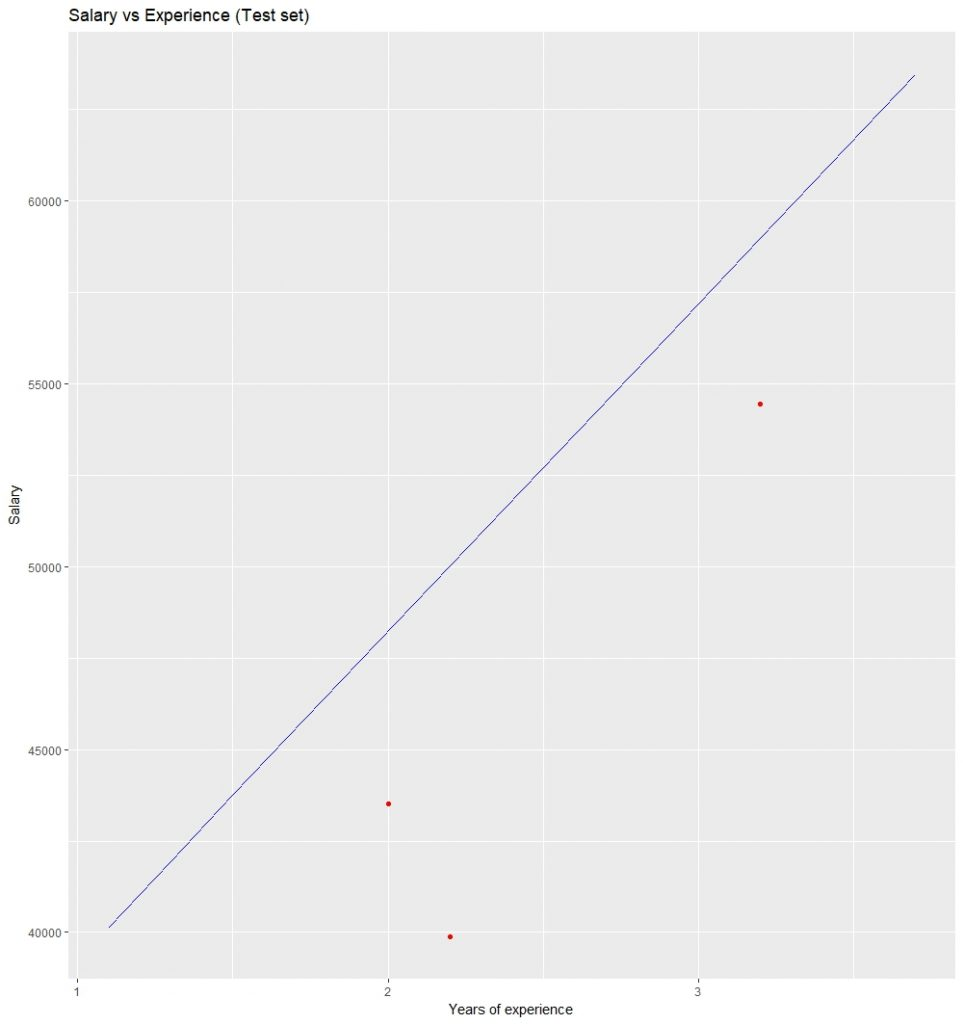## Unsupervised Learning

R – Unsupervised learning is the training of machines using information that is neither classified nor labeled and allowing the algorithm to act on that information without guidance. Here the task of the machine is to group unsorted information according to similarities, patterns, and differences without any prior training of data. Unlike supervised learning, no teacher is provided that means no training will be given to the machine. Therefore the machine is restricted to finding the hidden structure in unlabeled data by our-self. Unsupervised learning is classified into two categories of algorithms:

• Clustering: A clustering problem is where you want to discover the inherent groupings in the data, such as grouping customers by purchasing behavior.
• Association: An association rule learning problem is where you want to discover rules that describe large portions of your data, such as people that buy X also tend to buy Y.

### Types

Clustering:

1. Exclusive (partitioning)
2. Agglomerative
3. Overlapping
4. Probabilistic

Clustering Types:

1. Hierarchical clustering
2. K-means clustering
3. K-NN (k nearest neighbors)
4. Principal Component Analysis
5. Singular Value Decomposition
6. Independent Component Analysis

### Implement in k-means clustering in R

Let’s implement one of the very popular Unsupervised Learning i.e K-means clustering in R programming. K means clustering in R Programming is an Unsupervised Non-linear algorithm that clusters data based on similarity or similar groups. It seeks to partition the observations into a pre-specified number of clusters. Segmentation of data takes place to assign each training example to a segment called a cluster. In the unsupervised algorithm, high reliance on raw data is given with large expenditure on manual review for review of relevance is given. It is used in a variety of fields like Banking, healthcare, retail, Media, etc.

Syntax: kmeans(x, centers = 3, nstart = 10)

Where:

• x is numeric data
• centers is the pre-defined number of clusters
• the k-means algorithm has a random component and can be repeated nstart times to improve the returned model

Example:

## R

 `# Installing Packages``install.packages``(``"ClusterR"``)``install.packages``(``"cluster"``)` `# Loading package``library``(ClusterR)``library``(cluster)` `# Loading data``data``(iris)``   ` `# Structure ``str``(iris)` `# Removing initial label of``# Species from original dataset``iris_1 <- iris[, -5]` `# Fitting K-Means clustering Model``# to training dataset``set.seed``(240) ``# Setting seed``kmeans.re <- ``kmeans``(iris_1, centers = 3,``                            ``nstart = 20)``kmeans.re` `# Cluster identification for``# each observation``kmeans.re\$cluster` `# Confusion Matrix``cm <- ``table``(iris\$Species, kmeans.re\$cluster)``cm` `# Model Evaluation and visualization``plot``(iris_1[``c``(``"Sepal.Length"``, ``"Sepal.Width"``)])``plot``(iris_1[``c``(``"Sepal.Length"``, ``"Sepal.Width"``)],``            ``col = kmeans.re\$cluster)``plot``(iris_1[``c``(``"Sepal.Length"``, ``"Sepal.Width"``)],``               ``col = kmeans.re\$cluster,``               ``main = ``"K-means with 3 clusters"``)` `# Plotiing cluster centers``kmeans.re\$centers``kmeans.re\$centers[, ``c``(``"Sepal.Length"``,``                      ``"Sepal.Width"``)]` `# cex is font size, pch is symbol``points``(kmeans.re\$centers[, ``c``(``"Sepal.Length"``,``                             ``"Sepal.Width"``)],``       ``col = 1:3, pch = 8, cex = 3)` `# Visualizing clusters``y_kmeans <- kmeans.re\$cluster``clusplot``(iris_1[, ``c``(``"Sepal.Length"``, ``"Sepal.Width"``)],``                      ``y_kmeans,``                      ``lines = 0,``                      ``shade = ``TRUE``,``                      ``color = ``TRUE``,``                      ``labels = 2,``                      ``plotchar = ``FALSE``,``                      ``span = ``TRUE``,``                      ``main = ``paste``(``"Cluster iris"``),``                      ``xlab = ``'Sepal.Length'``,``                      ``ylab = ``'Sepal.Width'``)`

Output:

• Model kmeans_re: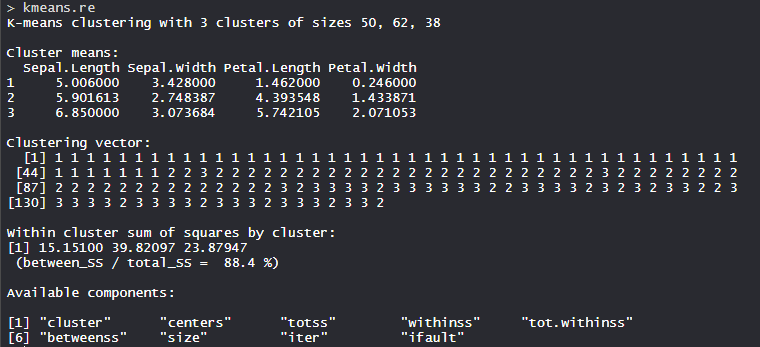The 3 clusters are made which are of 50, 62, and 38 sizes respectively. Within the cluster, the sum of squares is 88.4%.

• Cluster identification: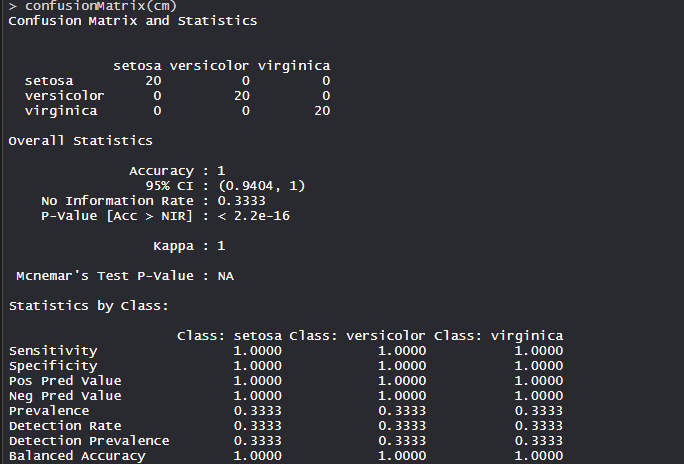The model achieved an accuracy of 100% with a p-value of less than 1. This indicates the model is good.

• Confusion Matrix: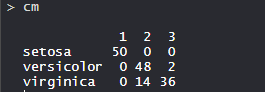So, 50 Setosa are correctly classified as Setosa. Out of 62 Versicolor, 48 Versicolor are correctly classified as Versicolor, and 14 are classified as virginica. Out of 36 virginica, 19 virginica are correctly classified as virginica and 2 are classified as Versicolor.

• K-means with 3 clusters plot: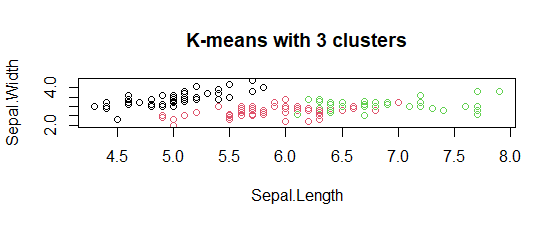The model showed 3 cluster plots with three different colors and with Sepal.length and with Sepal.width.

• Plotting cluster centers: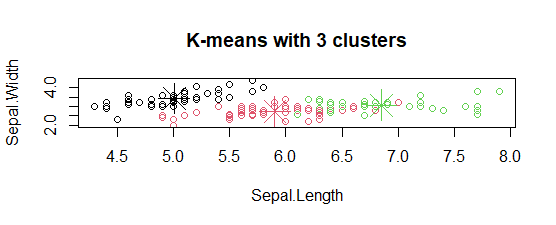In the plot, centers of clusters are marked with cross signs with the same color of the cluster.

• Plot of clusters: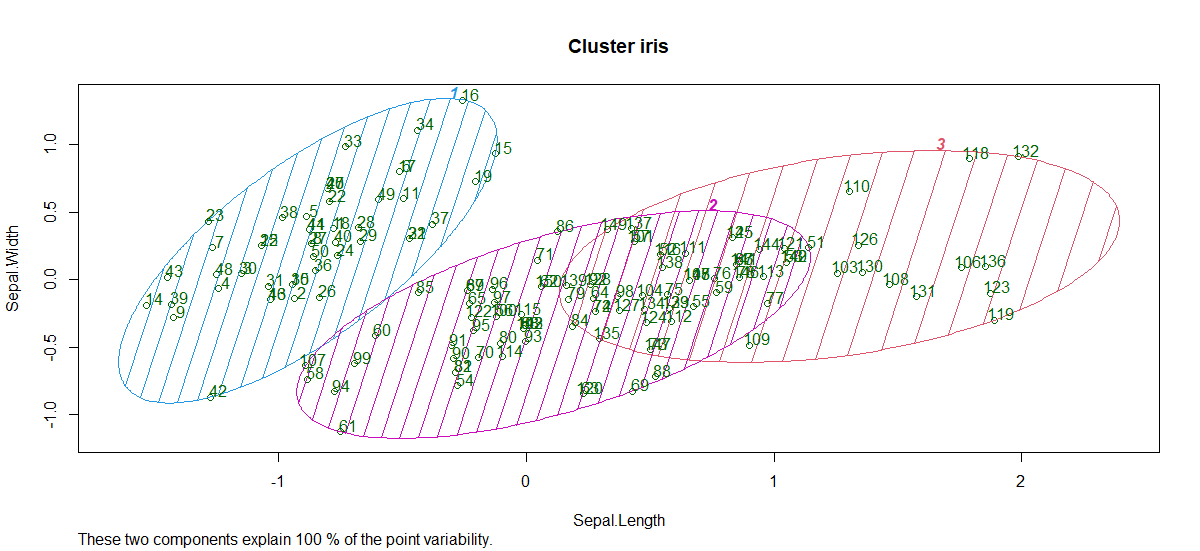So, 3 clusters are formed with varying sepal length and sepal width.

My Personal Notes arrow_drop_up